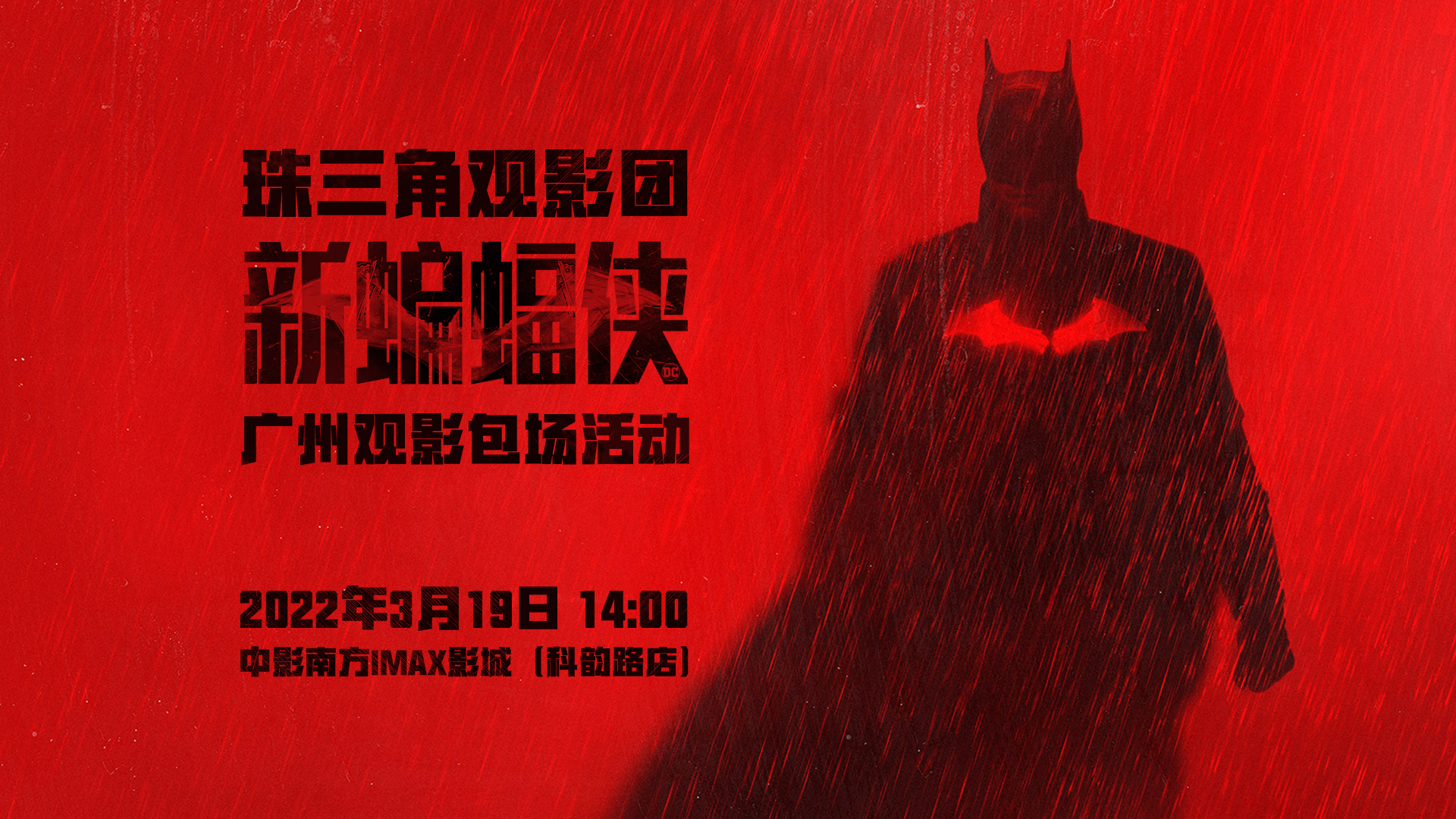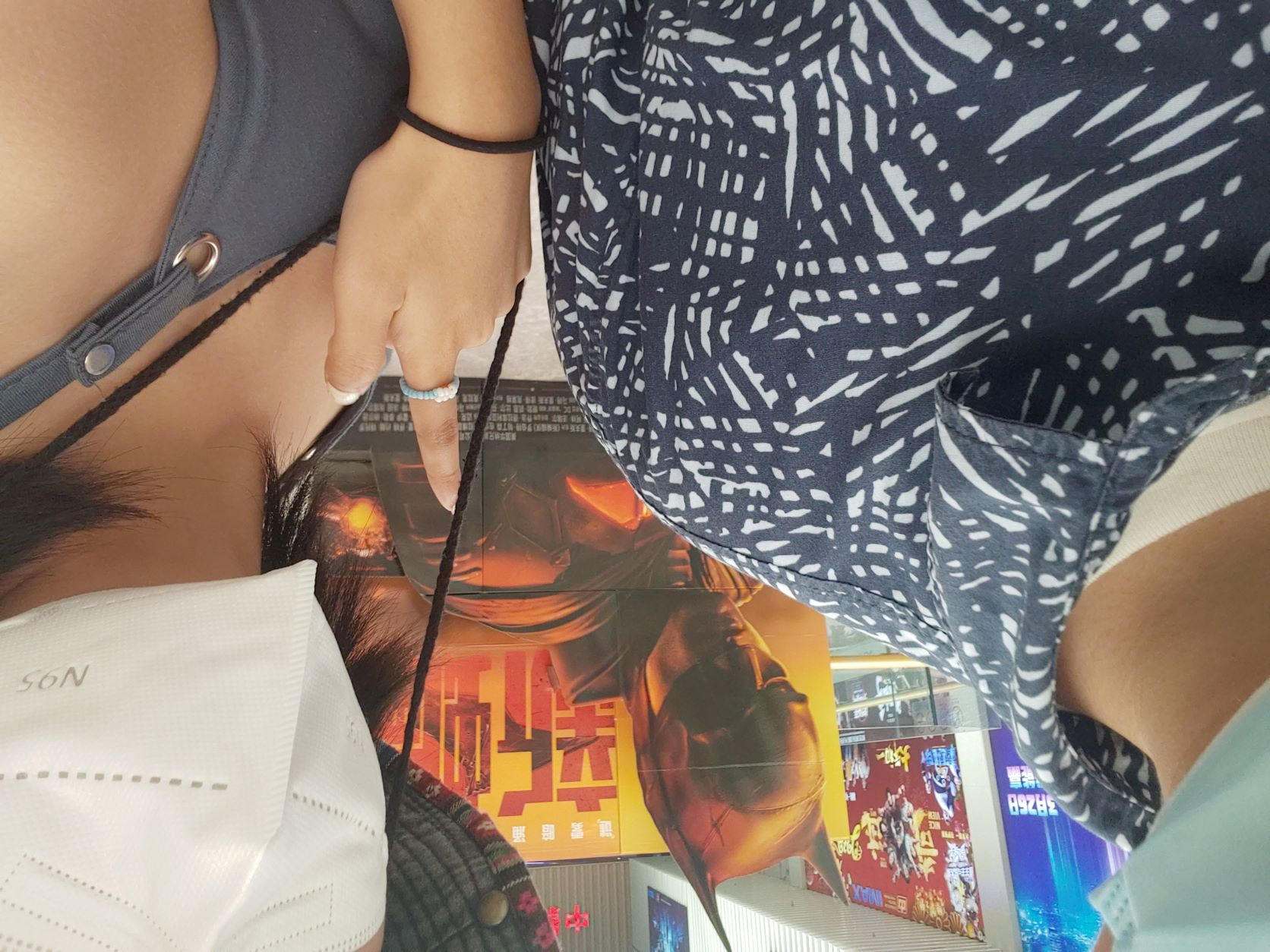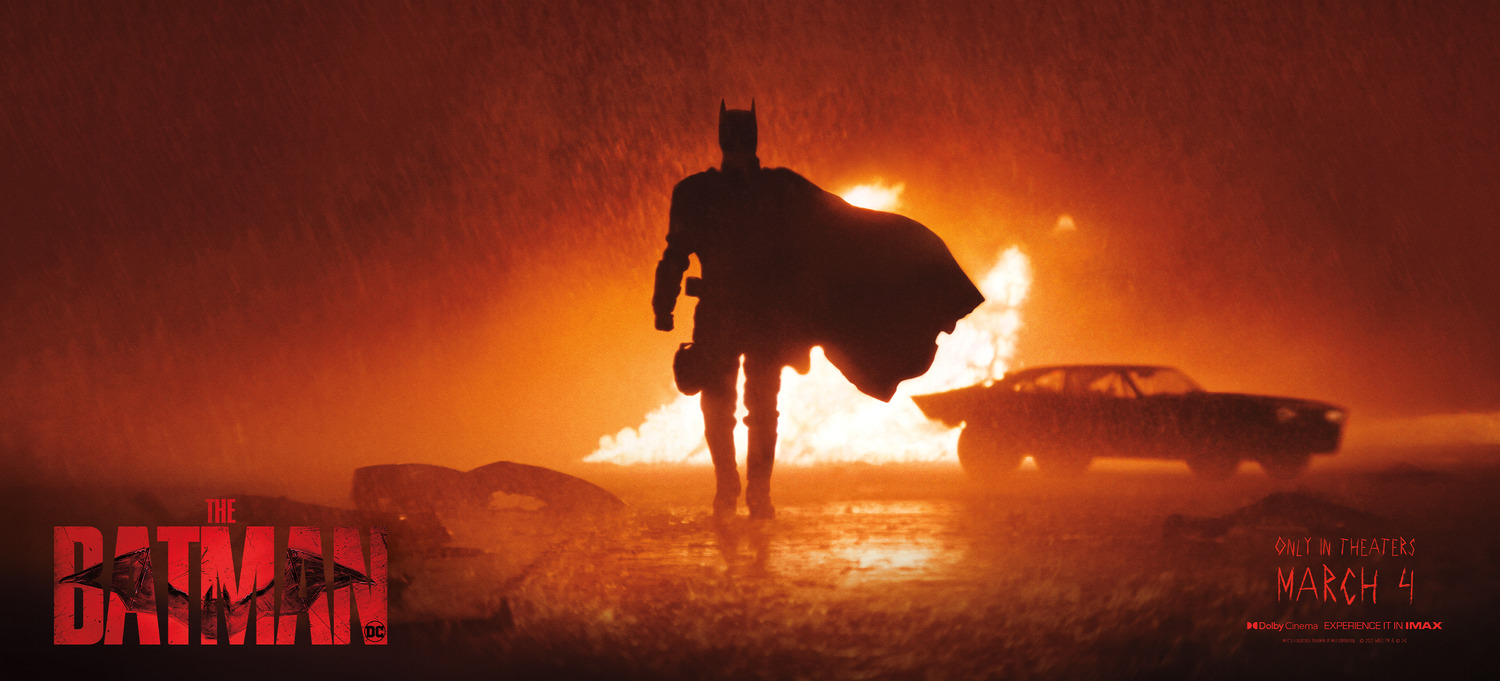# 第二年的《新蝙蝠侠》self.__wrap_n=self.__wrap_n||(self.CSS&&CSS.supports("text-wrap","balance")?1:2);self.__wrap_b=(e,n,t)=>{t=t||document.querySelector(`[data-br="\${e}"]`);let r=t.parentElement,o=f=>t.style.maxWidth=f+"px";t.style.maxWidth="";let c=r.clientWidth,a=r.clientHeight,s=c/2-.25,i=c+.5,p;if(c){for(o(s),s=Math.max(t.scrollWidth,s);s+1<i;)p=Math.round((s+i)/2),o(p),r.clientHeight===a?i=p:s=p;o(i*n+c*(1-n))}t.__wrap_o||(typeof ResizeObserver!="undefined"?(t.__wrap_o=new ResizeObserver(()=>{self.__wrap_b(0,+t.dataset.brr,t)})).observe(r): false&&0)};self.__wrap_n!=1&&self.__wrap_b(":Rarbb9mba:",1)## #前言## #电影## #角色## #总结